# Colleges with the highest SAT scores in South Carolina

Top 10 colleges in South Carolina with the highest SAT scores
Looking for the colleges with the highest SAT scores in South Carolina? Well you're in luck! We've compiled a national college database and have created a list of the top 10 universities with the highest SAT scores in South Carolina. These are the schools whose applicants had the highest average SAT scores in South Carolina. And, since these tests are meant to determine academic prowess, they are arguably the schools with the most academically proficient students. You could even say these are the best colleges in South Carolina. We also include each college's ACT scores and acceptance rate so that you can see where you would have the easiest or hardest time getting in. Read on to find out more.

## Clemson University SAT scores

The average SAT score for Clemson University is 1310.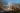The average SAT score of 1310 breaks down into:

• SAT math: 660

The average ACT score for Clemson University is 30 and their acceptance rate is 47%.

## Furman University SAT scores

The average SAT score for Furman University is 1309.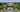The average SAT score of 1309 breaks down into:

• SAT math: 650

The average ACT score for Furman University is 29 and their acceptance rate is 61.1%.

## University of South Carolina SAT scores

The average SAT score for University of South Carolina is 1275.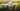The average SAT score of 1275 breaks down into:

• SAT math: 635

The average ACT score for University of South Carolina is 28 and their acceptance rate is 68.6%.

## Wofford College SAT scores

The average SAT score for Wofford College is 1270.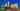The average SAT score of 1270 breaks down into:

• SAT math: 630

The average ACT score for Wofford College is 29 and their acceptance rate is 63.6%.

## College of Charleston SAT scores

The average SAT score for College of Charleston is 1166.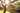The average SAT score of 1166 breaks down into:

• SAT math: 570

The average ACT score for College of Charleston is 25 and their acceptance rate is 79.3%.

## Anderson University South Carolina SAT scores

The average SAT score for Anderson University South Carolina is 1141.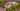The average SAT score of 1141 breaks down into:

• SAT math: 560

The average ACT score for Anderson University South Carolina is 23 and their acceptance rate is 79.1%.

## Presbyterian College SAT scores

The average SAT score for Presbyterian College is 1140.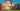The average SAT score of 1140 breaks down into:

• SAT math: 570

The average ACT score for Presbyterian College is 24 and their acceptance rate is 63%.

## The Citadel Military College of South Carolina SAT scores

The average SAT score for The Citadel Military College of South Carolina is 1118.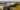The average SAT score of 1118 breaks down into:

• SAT math: 560

The average ACT score for The Citadel Military College of South Carolina is 23 and their acceptance rate is 81.1%.

## Coastal Carolina University SAT scores

The average SAT score for Coastal Carolina University is 1106.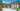The average SAT score of 1106 breaks down into:

• SAT math: 550

The average ACT score for Coastal Carolina University is 22 and their acceptance rate is 69.6%.

## North Greenville University SAT scores

The average SAT score for North Greenville University is 1105.The average SAT score of 1105 breaks down into:

• SAT math: 570